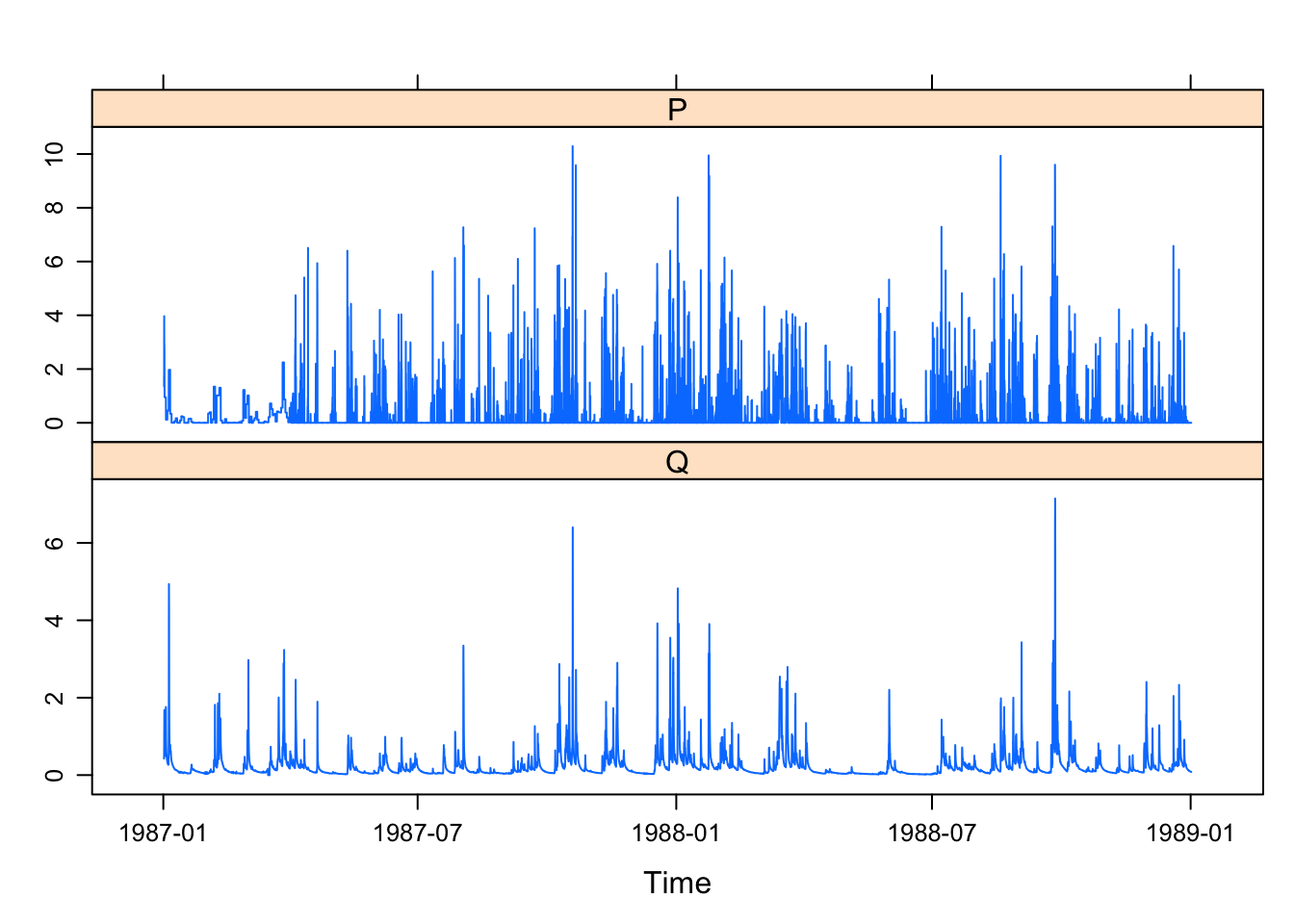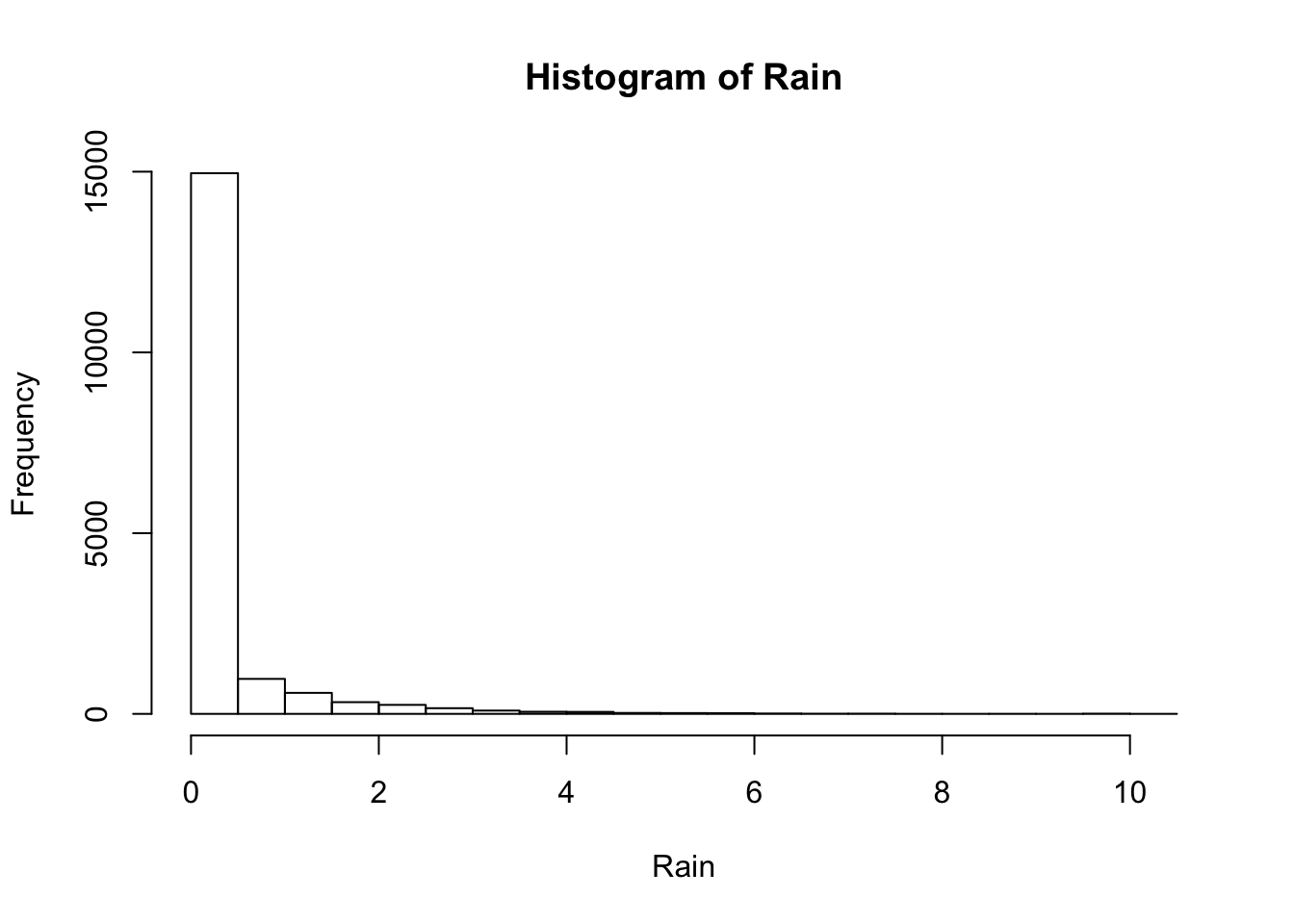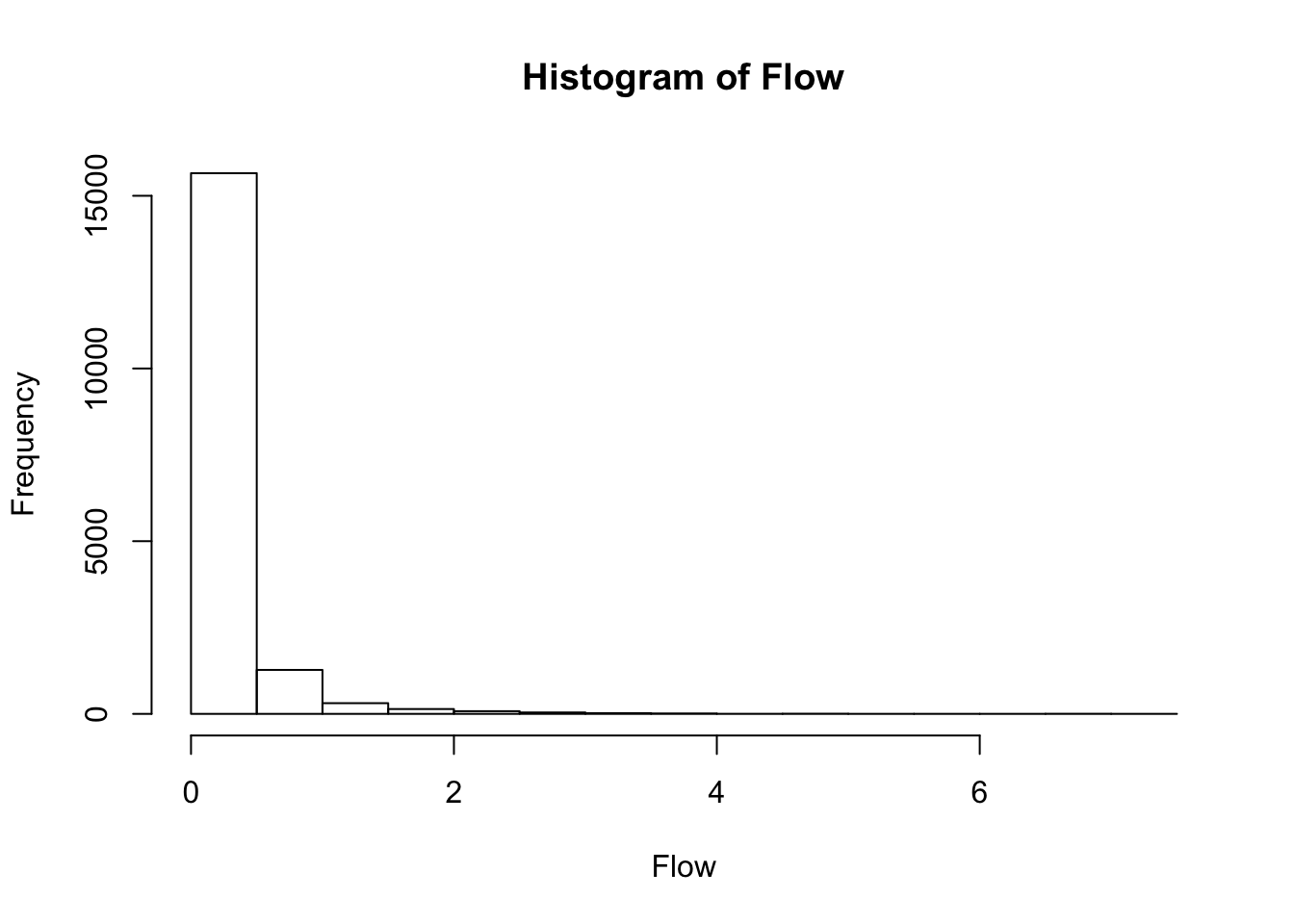# 1 Introduction

We are going to use a dataset from a river catchment area in Wales, included in the Hydrological Model Assessment and Development (hydromad http://hydromad.catchment.org) package. This upland catchment is the source of the River Wye, which is a significant river that occasionally floods in towns further downstream.

## 1.1 Aim

Create a forecast model to predict streamflow in the catchment for input rainfall. This model could be used for early warning of flooding downstream, or for designing hydraulic structures in the region.

# 2 Getting started

## 2.1 R and Rstudio versions

This practical works with R version 3.3.1 and RStudio version 1.0.44. The University ‘additional software’ is out of date and, if used, this markdown code fails to run. If you are using your laptop, this is not an issue, so skip to next section. If you are using a University workstation, then you need to download these two files:

Install R3.3.2 by running the .exe file, accepting the defaults. RStudio-1.0.44.zip can simply be moved to your ‘my documents’ folder and te hn unzipped.
To run RStudio, navigate in Windows Explorer to mydocuments-1.0.44and click on rstudio.exe.

## 2.2 Install packages

``````# This is a function to install any packages that cannot be loaded
# (Might be useful for coursework etc.)

my_required_packages <- function(x,y){
for( i in x ){
#  require returns TRUE if it was able to load package
if( ! require( i , character.only = TRUE ) ){
#  If package was not able to be loaded then re-install
install.packages( i , repos=y ,
#type="win.binary" , comment out so runs on OS X etc
quiet=TRUE , dependencies = TRUE , verbose = FALSE )
#  Load package after installing
require( i , character.only = TRUE, quietly = TRUE )
}
}
}``````

``````# call the function my_required_packages
my_required_packages(c("chron", "data.table", "zoo" , "latticeExtra", "polynom", "car", "Hmisc","reshape","DEoptim", "astsa", "pracma", "fractal", "tseries"),"http://cran.rstudio.com/")``````

Now install hydromad package:

``````# call the function my_required_packages

## 2.3 Load data

The data consists of two time series: rainfall (P) and streamflow data (Q). Both are in units of mm/hour. For stream flow, the flow rate has been divided by the catchment area; the rainfall has been averaged over the whole catchment area which is 10.6 km2.

``````data(Wye)

# Create a 'zoo' time series object
Wye<-zoo(coredata(Wye),seq(start(Wye),end(Wye),by="hours"))
summary(Wye)``````
``````##      Index                           P                 Q
##  Min.   :1987-01-01 12:00:00   Min.   : 0.0000   Min.   :0.0000
##  1st Qu.:1987-07-03 05:45:00   1st Qu.: 0.0000   1st Qu.:0.0628
##  Median :1988-01-01 23:30:00   Median : 0.0000   Median :0.1276
##  Mean   :1988-01-01 23:30:00   Mean   : 0.2827   Mean   :0.2430
##  3rd Qu.:1988-07-02 17:15:00   3rd Qu.: 0.1451   3rd Qu.:0.2683
##  Max.   :1989-01-01 11:00:00   Max.   :10.2922   Max.   :7.1485``````
``head(Wye)``
``````##                          P       Q
## 1987-01-01 12:00:00 1.7022 0.42625
## 1987-01-01 13:00:00 1.3528 0.49921
## 1987-01-01 14:00:00 1.8834 0.62421
## 1987-01-01 15:00:00 3.9669 0.93089
## 1987-01-01 16:00:00 3.0246 1.24750
## 1987-01-01 17:00:00 2.9156 1.52210``````
``tail(Wye)``
``````##                     P      Q
## 1989-01-01 06:00:00 0 0.0837
## 1989-01-01 07:00:00 0 0.0835
## 1989-01-01 08:00:00 0 0.0828
## 1989-01-01 09:00:00 0 0.0828
## 1989-01-01 10:00:00 0 0.0821
## 1989-01-01 11:00:00 0 0.0810``````
``````#Extract the core data from 'zoo' time series object
Rain<-coredata(Wye\$P)
Flow<-coredata(Wye\$Q)
t_start<-start(Wye)
t_end<-end(Wye)
t<-seq(t_start,t_end,by = "hour")``````

# 3 Inspecting data

## 3.1 Basic plots

Create a ‘run series plot’ (plot variables against time on the x-axis) and histograms of the variables

``````#plot.zoo(Wye, main = "River Wye Rainfall (P) and Streamflow (Q) in mm/h", xlab="Date")
xyplot(Wye)````````hist(Rain)````````#hist(Wye\$Q)
hist(Flow)``````### 3.1.1 Q. What are the average monthly rainfall figures?

``````P_mon=aggregate(Wye\$P, as.yearmon, FUN = sum)
aggregate(P_mon,function(x) cycle(as.yearmon(x)),FUN=mean)``````
``````##        1        2        3        4        5        6        7        8
## 168.1717 188.1225 314.2933 113.9667 141.0738 119.4974 214.8972 198.9812
##        9       10       11       12
## 254.4804 270.8295 190.6278 220.7620``````

### 3.1.2 Q. What is the “run-off ratio” (sum of streamflow)/(sum of rainfall)? Does this make sense?

``sum(Flow)/sum(Rain)``
``##  0.8595798``

### 3.1.3 Task: For the streamflow data, log-transform the variable and plot it against time

``plot.ts(as.Date(as.POSIXct(t)),log(Flow), main = "River Wye Streamflow Log(Q in mm/h)", xlab="Date")``